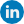# IBM SPSS software

SPSS standing for “Statistical Package for the Social Sciences” is a computer software widely used for statistical analysis in the social sciences.In addition to statistical analysis, this software facilitates data management and documentation.

The use of SPSS involves three general stages, namely importing data into the software, selecting the type of analysis, and obtaining the output

Generally, SPSS software has the following applications:

• Preparation of statistical summaries, such as graphs, tables, and statistics.
• Preparation of different tables, such as tables of frequency, cumulative frequency, and percentage of frequency
• Calculation of statistical distributions, including discrete and continuous distributions
• Calculation of mathematical functions, such as absolute value, sign function, logarithms, and trigonometric functions
• Provision of a variety of statistical designs
• Implementation of one-way and two-way analyses of variance, multivariate analysis, and analysis of covariance
• Implementation of time series analysis
• Creation of random and continuous data
• Calculation of a variety of descriptive statistics
• Implementation of tests related to the comparison of means between two or more independent and dependent variables
• Facilitation of exchanging information with other software
• Processing different types of regressions## 3 thoughts on “IBM SPSS software”

•JamesDub says:

IBM SPSS Statistics is the world’s leading statistical software used to solve business and research problems by means of ad-hoc analysis, hypothesis testing, geospatial analysis and predictive analytics. Organizations use IBM SPSS Statistics to understand data, analyze trends, forecast and plan to validate assumptions and drive accurate conclusions.

•furtdsolinopv says:

As I website owner I think the content here is really excellent, regards for your efforts.

•Sarah says:

Thank you